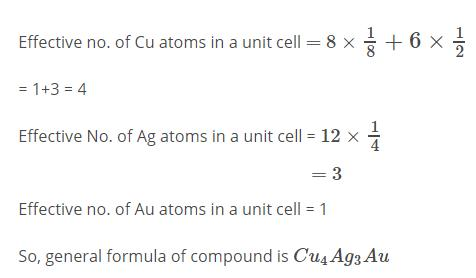Courses

# Test: Solid State- 1

## 20 Questions MCQ Test Physical Chemistry | Test: Solid State- 1

Description
This mock test of Test: Solid State- 1 for Chemistry helps you for every Chemistry entrance exam. This contains 20 Multiple Choice Questions for Chemistry Test: Solid State- 1 (mcq) to study with solutions a complete question bank. The solved questions answers in this Test: Solid State- 1 quiz give you a good mix of easy questions and tough questions. Chemistry students definitely take this Test: Solid State- 1 exercise for a better result in the exam. You can find other Test: Solid State- 1 extra questions, long questions & short questions for Chemistry on EduRev as well by searching above.
QUESTION: 1

### Which of the units given can be used to build the structure of covalent crystal, diamond:

Solution:

Diamond and Graphite, Two allotropes of carbon are covalent network solids which differ in the bonding geometry of the carbon atoms.

In diamond, the bonding occurs in the tetrahedral geometry, while in graphite the carbons bond with each other in the trigonal planar arrangement. This difference accounts for the drastically different appearance and properties of these two forms of carbon.

QUESTION: 2

### Which of the following expressions is true in case of a sodium chloride unit cell?

Solution:

In face-centered cubic system, the inter ionic distance is given by rc + ra = a/2

rc = Radius of the cation

ra = Radius of the Anion.

QUESTION: 3

### Solid CO2 is an example of the crystal type:

Solution:

Dry ice, or solid carbon dioxide, is a perfect example of a molecular solid. The van der Waals forces holding the CO2 molecules together are weak enough that dry ice sublimes - it passes directly from the solid to the gas phase - at -78oC.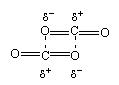QUESTION: 4

Name of the crystal class for which all the four types of unit cells are possible:

Solution: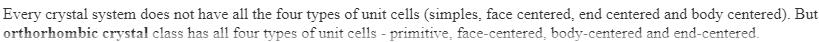QUESTION: 5

A metal crystallizes with a face-centered cubic lattice. The edge of the unit cell is 408 pm. The diameter of the metal atom is

Solution: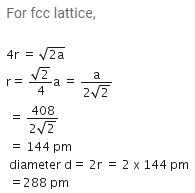QUESTION: 6

Which of the following is a molecular crystal?

Solution:

Molecular crystals are substances that have relatively weak intermolecular binding, such as Dry Ice (solidified carbon dioxide), solid forms of the rare gases (e.g., argon, krypton, and xenon), and crystals of numerous organic compounds.

QUESTION: 7

An element exists as hexagonal close packed structure as well as cubic closed packed structure. In which case the element would have higher density:

Solution:

The density of the element will be same in both the cases as the two structures will have the same coordination number and hence the same packing fraction.

QUESTION: 8

The molar volume of KCl and NaCl are 37.46 ml and 27.94 ml. The ratio of unit cell edges of the two crystals is:

Solution:

Molar volume =N4/3πr³ where N is Avogadro number ,

Now Molar volume of KCl is 37.46cm³ So N4/3πR³ = 37.46 cm³

On solving this gives R=2.458 x 10-8 cm

And for NaCl by using the same formula it gives R=2.22 x 10-8 cm

Now the ratio of edge length must be equal to ratio of radii as both belong to same crystal system so KCl/NaCl = 2.458/2.22 = 1.108

QUESTION: 9

A metal has a fcc lattice. The edge length of the unit cell is 404 pm. The density of the metal is 2.72 g cm-3. The molar mass of the metal is (NA Avogadro's constant= 6.02 x 1023 mol-1)

Solution:

Given, cell is fcc, So Z =4
Edge length, a = 404 pm = 4.04 x 10-8 cm
Density of metal, d = 2.72 g cm-3
NA = 6.02 x 1023 mol-1
Molar mass ofg the metal, M =?
We know that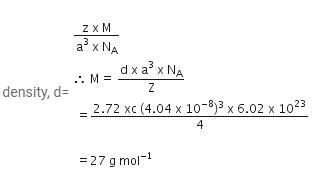QUESTION: 10

In a crystalline solid, anions B are arranged in CCP lattice and cations A occupy 50% of the octahedral voids and 50% of the tetrahedral voids. What is the formula of the solid:

Solution:

No of atoms in a CCP unit cell=similar to FCC=4

Therefore no of B atoms present=4

No of A atom=tetrahedral void and octohedral void(4+2)=6

The formula of the compound =A3​B2​

QUESTION: 11

If ‘a’ stand for the edge length of the cubic system: scc, bcc and fcc, then the ratio of the radii of the sphere in the system will be:

Solution: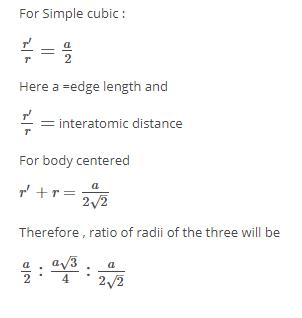QUESTION: 12

The packing efficiency of 2D square unit cell shown is: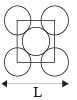Solution: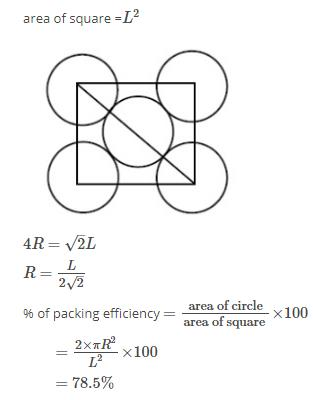QUESTION: 13

In which of the crystal lattice, alternate tetrahedral voids are occupied:

Solution:

In ZnS (zinc blends), Zn2+ ions are present in alternate tetrahedral voids.

QUESTION: 14

2D representation of the fcc unit cell is:

Solution:
QUESTION: 15

Na crystallizes in fcc lattice having edge length 500 pm. Mass of the Na particle is 4.151 × 10– 23g. The density of the lattice is:

Solution:
QUESTION: 16

Formula of the compound is: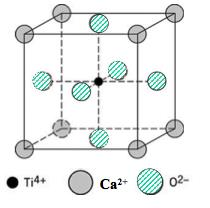Solution:

The centre atom will be considered as whole.

The face centre atom will be considered as half..

The corner atoms will be considered 1/8.

QUESTION: 17

Schottky defect generally appears in:

Solution:

Schottky defect is a type of point defect in a crystal lattice named after Walter H. Schottky. In non-ionic crystals it means a lattice valency defect. In ionic crystals, this point defect forms when oppositely charged ions leave their lattice sites, creating vacancies.

QUESTION: 18

Na2SeO4 and Na2SO4 show:

Solution:

Na2SO4 and Na2SeO4 are different substances possessing similar formula, they are called isomorphous systems

QUESTION: 19

The melting point of RbBr is 682°C, while that of NaF is 988°C. The principal reason that melting point of NaF is much higher than that of RbBr is that:

Solution:

In ionic compounds as internuclear distance incrreases, melting point decreases so as we move down the group, melting point decreases. So, as r+ ra  is greater for RbBr than for NaF so NaF have higher melting point.

QUESTION: 20

An alloy of Copper , Silver and Gold is found to have Copper constituting the C.C.P lattice. If Silver atoms occupy the edge centres and Gold is present at body centre , the alloy will have the formula:

Solution: